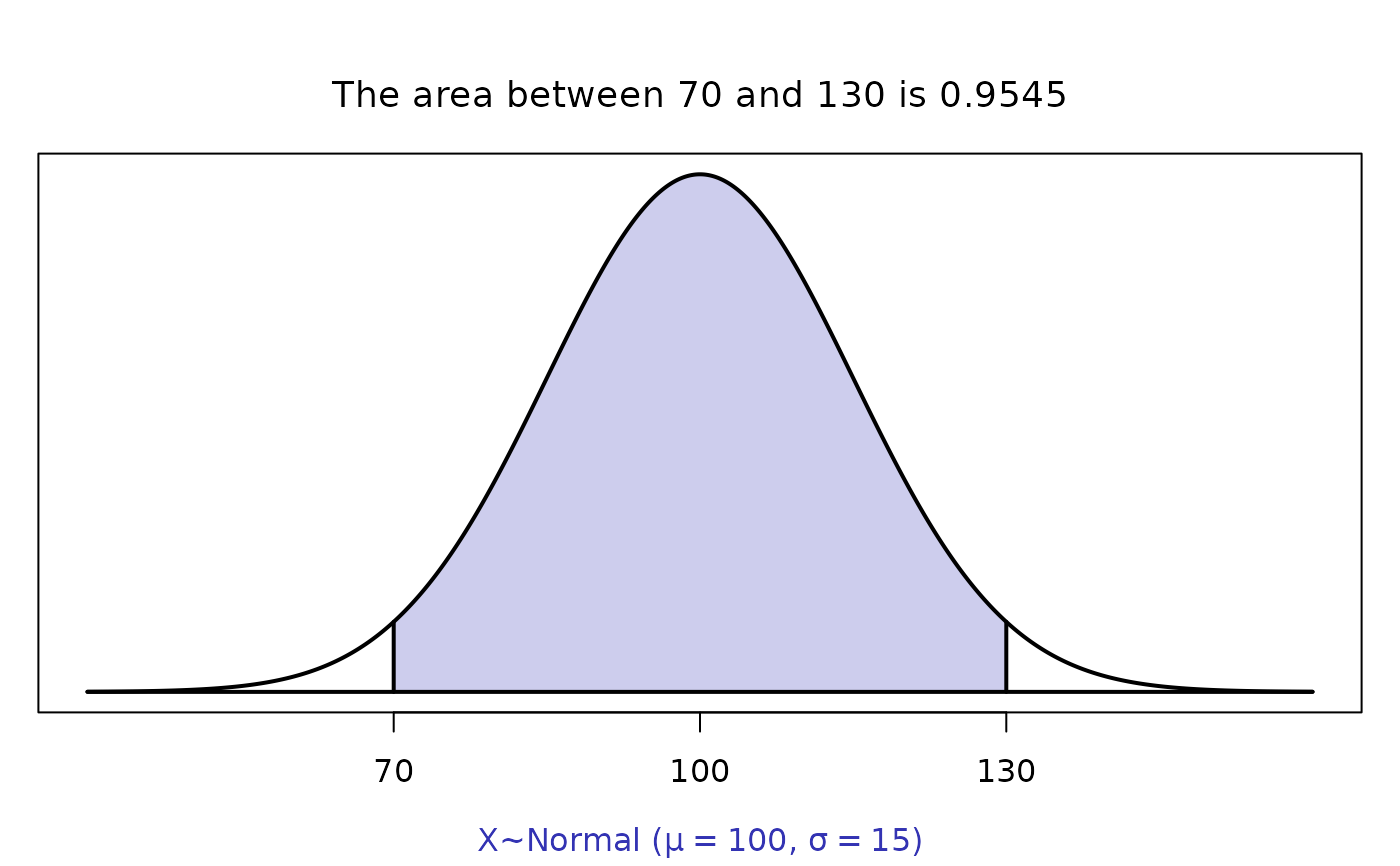Function that computes and draws the area between two user specified values in a user specified normal distribution with a given mean and standard deviation

normarea(lower = -Inf, upper = Inf, m, sig)

## Arguments

lower

the lower value

upper

the upper value

m

the mean for the population

sig

the standard deviation of the population

Alan T. Arnholt

## Examples


normarea(70, 130, 100, 15)# Finds and P(70 < X < 130) given X is N(100,15).